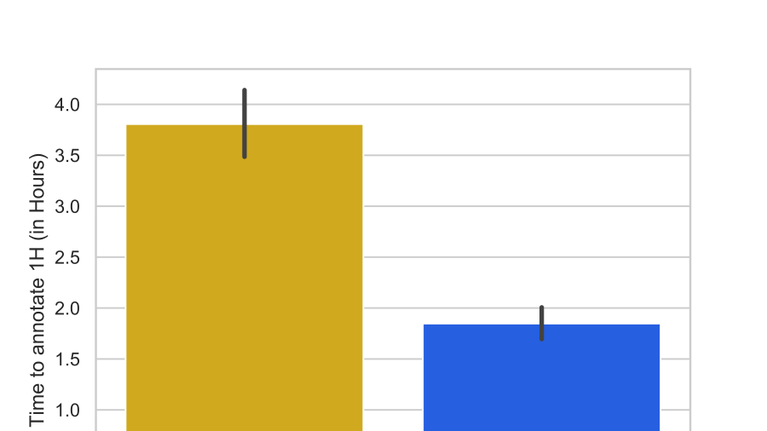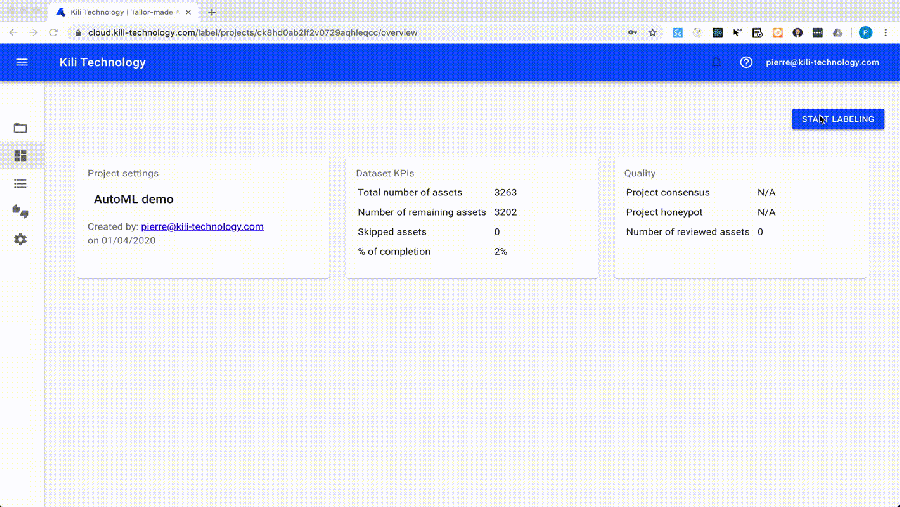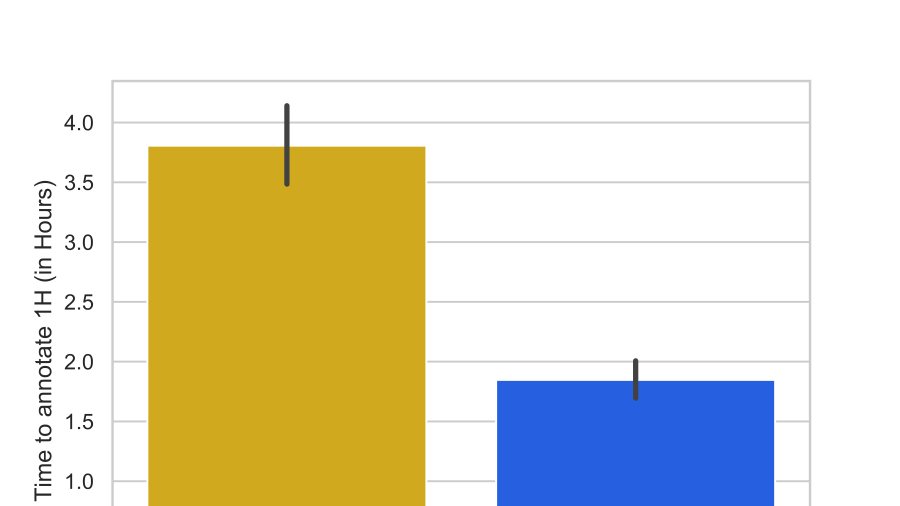• Products
• Solutions
• Company
• Resources
• Docs
• Pricing

# AutoML for fast annotation

This tutorial will help you understand that how automated machine learning (AutoML) is used to accelerate your labelling process.## AutoML for fast labeling with Kili Technology

This tutorial is taken from our recipes. You can find an executable version of the Jupyter notebook on Github.

In this tutorial, we will show how to use automated machine learning (AutoML) to accelerate labeling in Kili Technology. We will apply it in the context of text classification: given a tweet, I want to classify whether it is about a real disaster or not (as introduced in Kaggle NLP starter kit).

Why want to label more data when Kaggle often provides with a fully annotated training set and a testing set?

• Annotate the testing set in order to have more training data once you fine-tuned an algorithm (once you are sure you do not overfit). More data almost always means better scores in machine learning.

• As a data scientist, annotate data in order to get a feel of what data looks like and what ambiguities are.

But annotating data is a time-consuming task. So we would like to help you annotate faster by fully automating machine learning models thanks to AutoML. Here is what is looks like in Kili:For an overview of Kili, visit kili-technology.com. You can also check out Kili documentation.

The tutorial is divided into three parts:

1. AutoML

2. Integrate AutoML scikit-learn pipelines

3. Automating labeling in Kili Technology

## AutoML

Automated machine learning (AutoML) is described as the process of automating both the choice and training of a machine learning algorithm by automatically optimizing its hyperparameters.

There already exist many AutoML framework:

• H2O provides with an AutoML solution with both Python and R bindings

• autosklearn can be used for SKLearn pipelines

• TPOT uses genetic algorithms to automatically tune your algorithms

• fasttext has its own AutoML module to find the best hyperparameters

We will cover the use of autosklearn for automated text classification. autosklearn explores the hyperparameters grid as defined by SKLearn as a human would do it manually. Jobs can be run in parallel in order to speed up the exploration process. autosklearn can use either SMAC (Sequential Model-based Algorithm Configuration) or random search to select the next set of hyperparameters to test at each time.

Once AutoML automatically chose and trained a classifier, we can use this classifier to make predictions. Predictions can then be inserted into Kili Technology. When labeling, labelers first see predictions before labeling. For complex tasks, this can considerably speed up the labeling.

For instance, when annotating voice for automatic speech recognition, if you use a model that pre-annotates by transcribing speeches, you more than double annotation productivity:## Integrate AutoML scikit-learn pipelines

Specifically for text classification, the following pipeline retrieves labeled and unlabeled data from Kili, builds a classifier using AutoML and then enriches back Kili's training set:After retrieving data, TFIDF pre-processes text data by filtering out common words (such as the, a, etc) in order to make most important features stand out. These pre-processed features will be fed to a classifier.

Note: autosklearn runs better on Linux, so we recommend running code snippets inside a Docker image:

``````docker run --rm -it -p 10000:8888 -v /local/path/to/notebook/folder:/home/kili --entrypoint "/bin/bash" mfeurer/auto-sklearn

# cd /home/kili && jupyter notebook --ip=0.0.0.0 --port=8888 --allow-root

from sklearn.feature_extraction.text import CountVectorizer, TfidfVectorizer
from sklearn.feature_selection import SelectKBest, f_classif
from sklearn.metrics import accuracy_score

MIN_DOC_FREQ = 2
NGRAM_RANGE = (1, 2)
TOP_K = 20000
TOKEN_MODE = 'word'

def ngram_vectorize(train_texts, train_labels, val_texts):
tfidf_vectorizer_params = {
'ngram_range': NGRAM_RANGE,
'dtype': 'int32',
'strip_accents': 'unicode',
'decode_error': 'replace',
'analyzer': TOKEN_MODE,
'min_df': MIN_DOC_FREQ,
}

# Learn vocab from train texts and vectorize train and val sets

tfidf_vectorizer = TfidfVectorizer(**tfidf_vectorizer_params)
x_train = tfidf_vectorizer.fit_transform(train_texts)
x_val = tfidf_vectorizer.transform(val_texts)

# Select k best features, with feature importance measured by f_classif

selector = SelectKBest(f_classif, k=min(TOP_K, x_train.shape))
selector.fit(x_train, train_labels)
x_train = selector.transform(x_train).astype('float32')
x_val = selector.transform(x_val).astype('float32')

return x_train, x_val``````

Labeled data is split in train and test sets for validation. Then, autosklearn classifier is chosen and trained in a limited time.

``````from tempfile import TemporaryDirectory

# Un comment these lines if you are not running inside autosklearn container
# !conda install gxx_linux-64 gcc_linux-64 swig==3.0.12 --yes
# !pip install auto-sklearn

import autosklearn
import autosklearn.classification
from sklearn.model_selection import train_test_split

def automl_train_and_predict(X, y, X_to_predict):
x, x_to_predict = ngram_vectorize(
X, y, X_to_predict)
x_train, x_test, y_train, y_test = train_test_split(
x, y, test_size=0.2, random_state=42)

# Auto-tuning by autosklearn

per_run_time_limit=20,
seed=10)
cls.fit(x_train, y_train)
assert x_train.shape == x_to_predict.shape

# Performance metric

predictions_test = cls.predict(x_test)
print('Accuracy: {}'.format(accuracy_score(y_test, predictions_test)))

# Generate predictions

predictions = cls.predict(x_to_predict)
return predictions``````

## Automating labeling in Kili Technology

Let's now feed Kili data to the AutoML pipeline. For that you will need to create a new Text classification project. Assets are taken from Kaggle challenge Real or Not? NLP with Disaster Tweets. You can download them here.

Connect to Kili Technology using kili-playground (Kili's official Python SDK to interact with Kili API):

``````!pip install kili
from kili.authentication import KiliAuth
from kili.playground import Playground

api_endpoint = 'https://cloud.kili-technology.com/api/label/graphql'

playground = Playground(kauth)``````

Let's insert all assets into Kili. You can download the original unannotated test.csv directly on Kaggle.

``````import pandas as pd

content_array = []
external_id_array = []

for index, row in df.iterrows():
external_id_array.append(f'tweet_{index}')
content_array.append(row['text'])

playground.append_many_to_dataset(project_id=project_id,
content_array=content_array,
external_id_array=external_id_array,
is_honeypot_array=[False for _ in content_array],
status_array=['TODO' for _ in content_array],

Retrieve the categories of the first job that you defined in Kili interface. Learn here what interfaces and jobs are in Kili.

``````project = playground.projects(project_id=project_id)
assert 'jsonInterface' in project

json_interface = project['jsonInterface']
jobs = json_interface['jobs']
jobs_list = list(jobs.keys())
assert len(jobs_list) == 1, 'More than one job was defined in the interface'

job_name = jobs_list
job = jobs[job_name]
categories = list(job['content']['categories'].keys())
print(f'Categories are: {categories}')

``````

We continuously fetch assets from Kili Technology and apply AutoML pipeline. You can launch the next cell and go to Kili in order to label. After labeling a few assets, you'll see predictions automatically pop up in Kili!

Go here to learn in more details how to insert predictions into Kili.

``````import os
import time
import warnings

!pip install tqdm
from tqdm import tqdm

warnings.filterwarnings('ignore')

SECONDS_BETWEEN_TRAININGS = 60

def extract_train_for_auto_ml(job_name, assets, categories, train_test_threshold=0.8):
X = []
y = []
X_to_predict = []
ids_X_to_predict = []
for asset in assets:
x = asset['content']
labels = [l for l in asset['labels'] if l['labelType'] in ['DEFAULT', 'REVIEWED']]

# If no label, add it to X_to_predict

if len(labels) == 0:
X_to_predict.append(x)
ids_X_to_predict.append(asset['externalId'])

# Otherwise add it to training examples X, y

for label in labels:
jsonResponse = label['jsonResponse'][job_name]
is_empty_label = 'categories' not in jsonResponse or len(
jsonResponse['categories']) != 1 or 'name' not in jsonResponse['categories']
if is_empty_label:
continue
X.append(x)
y.append(categories.index(
jsonResponse['categories']['name']))

return X, y, X_to_predict, ids_X_to_predict

while True:
print('Export assets and labels...')
assets = playground.assets(project_id=project_id, first=100, skip=0) ## Remove that
X, y, X_to_predict, ids_X_to_predict = extract_train_for_auto_ml(job_name, assets, categories)

version = 0

if len(X) > 5:
print('AutoML is on its way...')
predictions = automl_train_and_predict(X, y, X_to_predict)
print('Inserting predictions to Kili...')
external_id_array = []
json_response_array = []

for i, prediction in enumerate(tqdm(predictions)):
json_response = {
job_name: {
'categories': [{
'name': categories[prediction],
'confidence':100
}]
}
}

external_id_array.append(ids_X_to_predict[i])
json_response_array.append(json_response)

# Good practice: version your model so you know the result of every model

playground.create_predictions(project_id=project_id,
external_id_array=external_id_array,
model_name_array=[f'automl-{version}']*len(external_id_array),
json_response_array=json_response_array)
print('Done.\n')

time.sleep(SECONDS_BETWEEN_TRAININGS)

version += 1``````

## Summary

In this tutorial, we accomplished the following:

We introduced the concept of AutoML as well as several of the most-used frameworks for AutoML. We demonstrated how to leverage AutoML to automatically create predictions in Kili. If you enjoyed this tutorial, check out the other Recipes for other tutorials that you may find interesting, including demonstrations of how to use Kili.

You can also visit the Kili website or Kili documentation for more info!